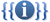Path of Exile Wiki

Please consider helping keep the wiki up to date. Check the to-do list of updates needed for version 3.14.0.

Game data exports will becoming later as the technical changes in addition to regular changes take some more time.Template documentation[view]  [history] [purge]

The {{math}} template is a wrapper for [itex] tag which is a part of the Extension:Math. The template is mainly used to change the default rendering colors (black text on white background) to custom ones that fit the color scheme of the wiki, but also brings additional functionality such as possibility of using another templates inside of [itex] tags and support for numbered equations.

For a detailed guide to LaTeX markup see Help:Displaying a formula.

Usage

Code {{math|2+3}} renders as:Parameters

The following parameters are available

Parameter Description
|formula= Use it if formula contains = sign.
|display= Display attribute with possible values:
• block: equation is rendered in its own paragraph and has indent
• inline: there will be no new paragraph and the operators will be rendered to consume only a small amount of vertical space
• not specified: no new paragraph and renders similar to block
|nr= Activates numbered equations. Don't forget to use unique numbers. Use {{EquationNote}} to reference the numbered equation.

Basic usage

For more examples, see Template:Math/testcases.

Display

 default The sum {{math |formula=\sum_{i=0}^\infty 2^{-i} }} converges to 2. The sumconverges to 2. block The sum {{math |display=block |formula=\sum_{i=0}^\infty 2^{-i} }} converges to 2. The sumconverges to 2. inline The sum {{math |display=inline |formula=\sum_{i=0}^\infty 2^{-i} }} converges to 2. The sumconverges to 2.

Numbered equations

The equations can be numbered using the nr parameter. The equation can then be referenced with {{EquationNote}}. For example:

{{math|nr=1|formula=
x^2 + y^2 + z^2 = 1
}}
Equation {{EquationNote|1}} shows the equation for an sphere centered around origo.(1)

Equation (1) shows the equation for a sphere centered around origo.

Combining with queried data

It is possible to query data with various parser functions such as #cargo_query, #ask and other parser functions such as #expr. Which helps making sure equations stay up date even if the game changes. For example:

{{#vardefine:life_leech_rate| {{Character query|maximum_life_leech_rate_%_per_minute}} }}
{{math|formula=
Maximum Leech Rate Per Minute / 60
=          {{#var:life_leech_rate}} / 60
= {{#expr: {{#var:life_leech_rate}} / 60 }}
}}

yields:Limitations

Error with multiple braces

Problem

Placing multiple opening/closing braces together (e.g. {{ or }}) if there are no closing/opening brackets, causes error. This happens because interpreter thinks they are a part of a template when in fact they are a part of a math markup. For example, the following code:

 {{math|formula= DR(A, D_{raw}) = {A \over A + 10 \times D_{raw}} }}

$163,141,109} DR(A, D_{raw$ }}

Solution

In order to prevent this either space braces:

 {{math|formula= DR(A, D_{raw}) = {A \over A + 10 \times D_{raw} } }}or use the following templates:

 {{math|formula= DR(A, D_{raw}) = {A \over A + 10 \times D_{raw{{))}} }}Absolute value denotation

Problem

Absolute values are usually denoted |x|. However using | in templates will result in the following issue.

 {{math|formula= |x| }}Solution

Use \vert or {{!}}:

 {{math|formula= \vert x\vert }}{{math|formula= {{!}}x{{!}} }}Template forces new line in lists

Problem

When using the template with unordered/ordered lists the template forces a new line.

 # The highest-level talisman is counted '''three''' times, {{Math|H}}. # The middle three talismans are counted '''twice''', {{Math|[M_1, M_2, M_3]}}. # The lowest-level talisman is counted '''once''', {{Math|L}}. The highest-level talisman is counted three times,. The middle three talismans are counted twice,. The lowest-level talisman is counted once,.

Solution

In order to prevent this use html lists.


1. The highest-level talisman is counted '''three''' times, {{Math|H}}.
2. The middle three talismans are counted '''twice''', {{Math|[M_1, M_2, M_3]}}.
3. The lowest-level talisman is counted '''once''', {{Math|L}}.
The highest-level talisman is counted three times,. The middle three talismans are counted twice,. The lowest-level talisman is counted once,.